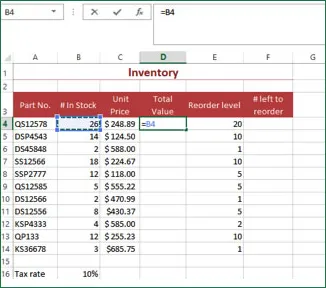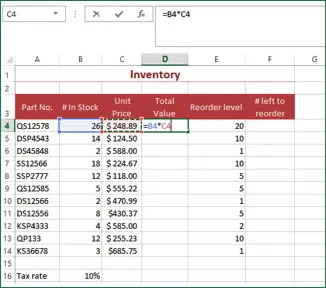# Building Formulas in Microsoft Excel

The backbone of Excel is its ability to perform calculations. There are two ways to set up calculations in Excel: using formulas or using functions. Formulas are mathematical expressions that you build yourself. You need to follow proper math principles in order to obtain the expected answer. Building the formula is simply a matter of combining the proper cell addresses with the correct operators in the right order. This module will explore how to build, edit, and copy formulas. This module will also explain the difference between relative and absolute references. Finally, this module will explain how to use the Status Bar to perform simple calculations. We will explore functions in the next module.

For instructor-led MS Excel training classes in Los Angeles call us on 888.815.0604.

#### The Math Basics of Excel

Review the different types of operators.

###### The Arithmetic operators are:

+ Plus Sign - Adds values
- Minus Sign - Subtracts values
* Asterisk - Multiplies values
/ Forward slash - Divides values
% Percent sign - Finds the percentage of a value
^ Caret - Exponentiation - Finds the exponential value

###### The Comparison operators are:

= Equals sign - Equates values
> Greater than sign - Indicates that one value is greater than the other
< Less than sign - Indicates that one value is less than the other
>= Greater than or equal to - Indicates that one value is greater than or equal to the other
<= Less than or equal to - Indicates that one value is less than or equal to the other
<> Not Equal - Indicates that values are not equal

Text concatenation allows you to combine text from different cells into a single piece of text. The operator is the & sign.

###### The reference operators combine a range of cells to use together in an operation. The reference operators are:

: Colon - A Range operator that produces a reference to all of the cells between the references on either side of the colon
, Comma - A Union operator that combines multiple range references
- An intersection operator that returns a reference to the cells common to the ranges in the formula

#### Building a Formula

To enter a formula to calculate the Total Value in the sample worksheet, use the following procedure.1. Select the Total Value column for the first product (cell D4).
2. Enter the = sign to begin the formula.
3. Select cell B4 to use it as the first value in the formula. Excel enters the reference as part of the formula.1. Enter the * sign.
2. Click on cell C4 to use it as the second value in the formula. Excel enters the references as part of the formula.
3. Press ENTER to complete the formula. Excel moves to the next row and performs the calculations in the formula.

The following illustration shows the answer to the calculation in the cell, and since the cell is active, you can see the formula in the Formula bar.

#### Group Excel training

Through our network of local trainers we deliver onsite group Microsoft Excel training right across the country. View our Excel training testimonials or obtain a quote for a customized Excel class.